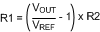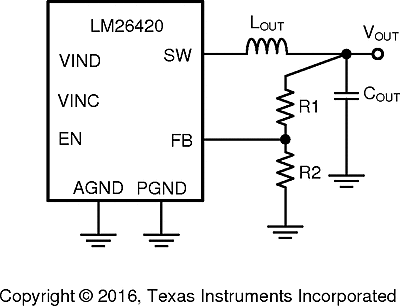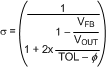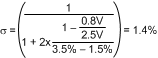SNVS579L February   2009  – May 2018

PRODUCTION DATA.

1. Features
2. Applications
3. Description
1.     Device Images
4. Revision History
5. Pin Configuration and Functions
6. Specifications
7. Detailed Description
1. 7.1 Overview
2. 7.2 Functional Block Diagram
3. 7.3 Feature Description
4. 7.4 Device Functional Modes
8. Application and Implementation
1. 8.1 Application Information
2. 8.2 Typical Applications
1. 8.2.1 LM26420X 2.2-MHz, 0.8-V Typical High-Efficiency Application Circuit
2. 8.2.2 LM26420X 2.2-MHz, 1.8-V Typical High-Efficiency Application Circuit
3. 8.2.3 LM26420X 2.2-MHz, 2.5-V Typical High-Efficiency Application Circuit
4. 8.2.4 LM26420Y 550 kHz, 0.8-V Typical High-Efficiency Application Circuit
5. 8.2.5 LM26420Y 550-kHz, 1.8-V Typical High-Efficiency Application Circuit
6. 8.2.6 LM26420Y 550-kHz, 2.5-V Typical High-Efficiency Application Circuit
9. Power Supply Recommendations
10. 10Layout
11. 11Device and Documentation Support
12. 12Mechanical, Packaging, and Orderable Information

#### 8.1.1 Programming Output Voltage

The output voltage is set using Equation 1 where R2 is connected between the FB pin and GND, and R1 is connected between VOUT and the FB pin. A good value for R2 is 10 kΩ. When designing a unity gain converter (VOUT = 0.8 V), R1 must be between 0 Ω and 100 Ω, and R2 must be on the order of 5 kΩ to 50 kΩ. 10 kΩ is the suggested value where R1 is the top feedback resistor and R2 is the bottom feedback resistor.

Equation 1.Equation 2. VREF = 0.80VFigure 29. Programming VOUT

To determine the maximum allowed resistor tolerance, use Equation 3:

Equation 3.where

• TOL is the set point accuracy of the regulator, is the tolerance of VFB.

Example:

VOUT = 2.5 V, with a setpoint accuracy of ±3.5%.

Equation 4.Choose 1% resistors. If R2 = 10 kΩ, then R1 is 21.25 kΩ.